Tangents and Normals (using Differentiation)

Chapter 6 Class 12 Application of Derivatives
Serial order wise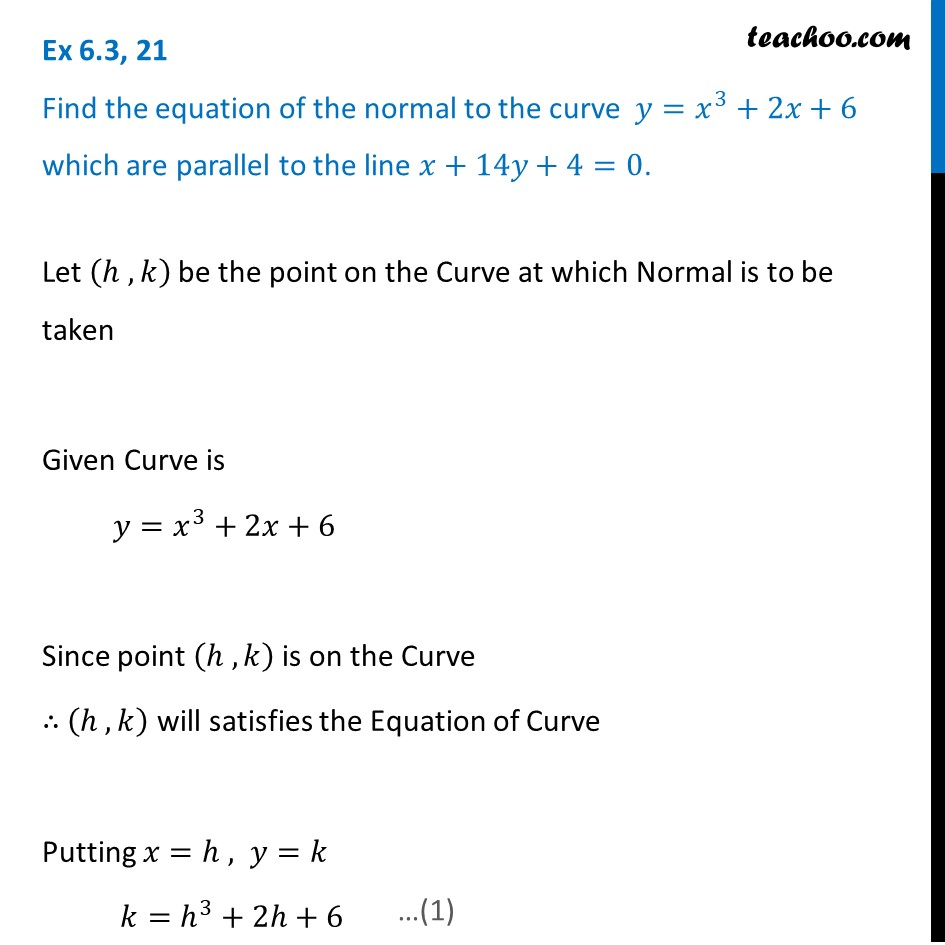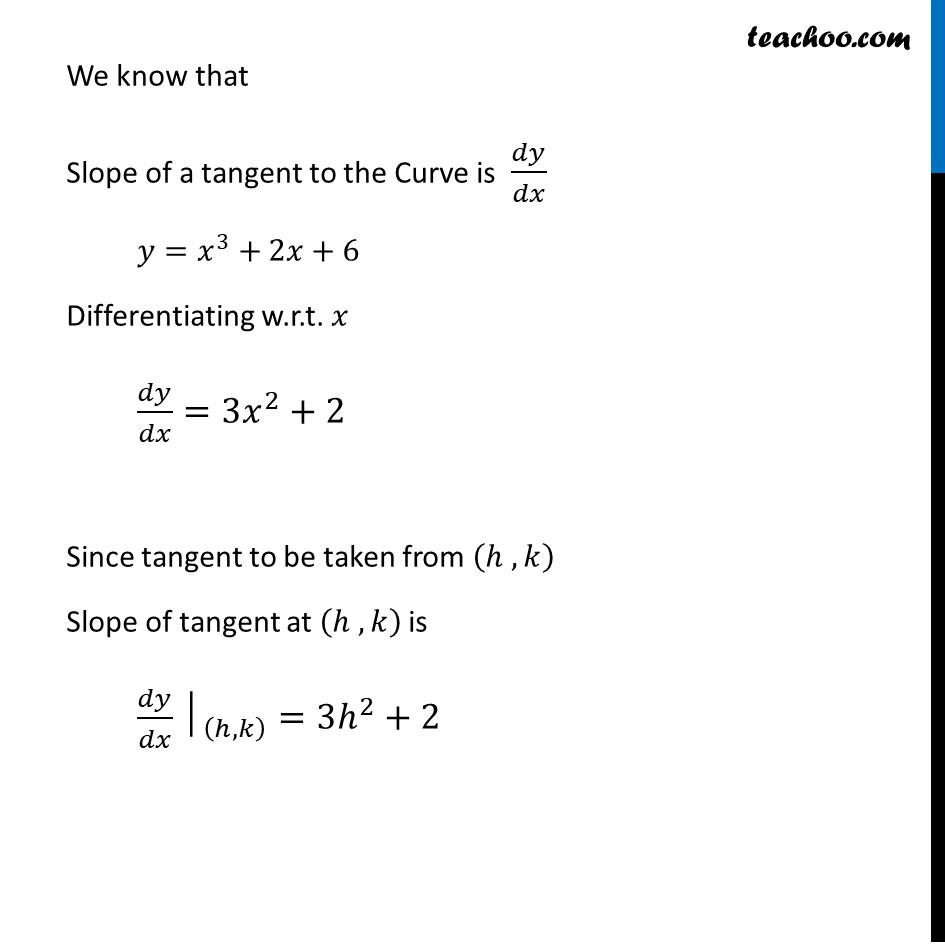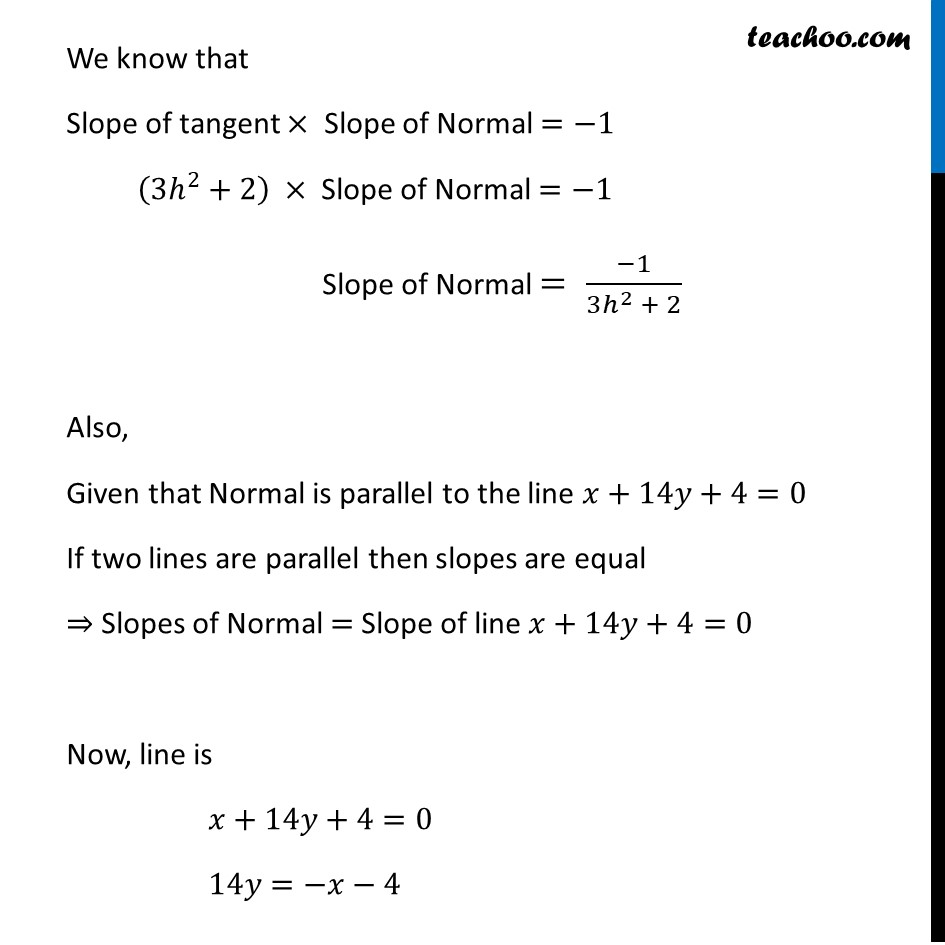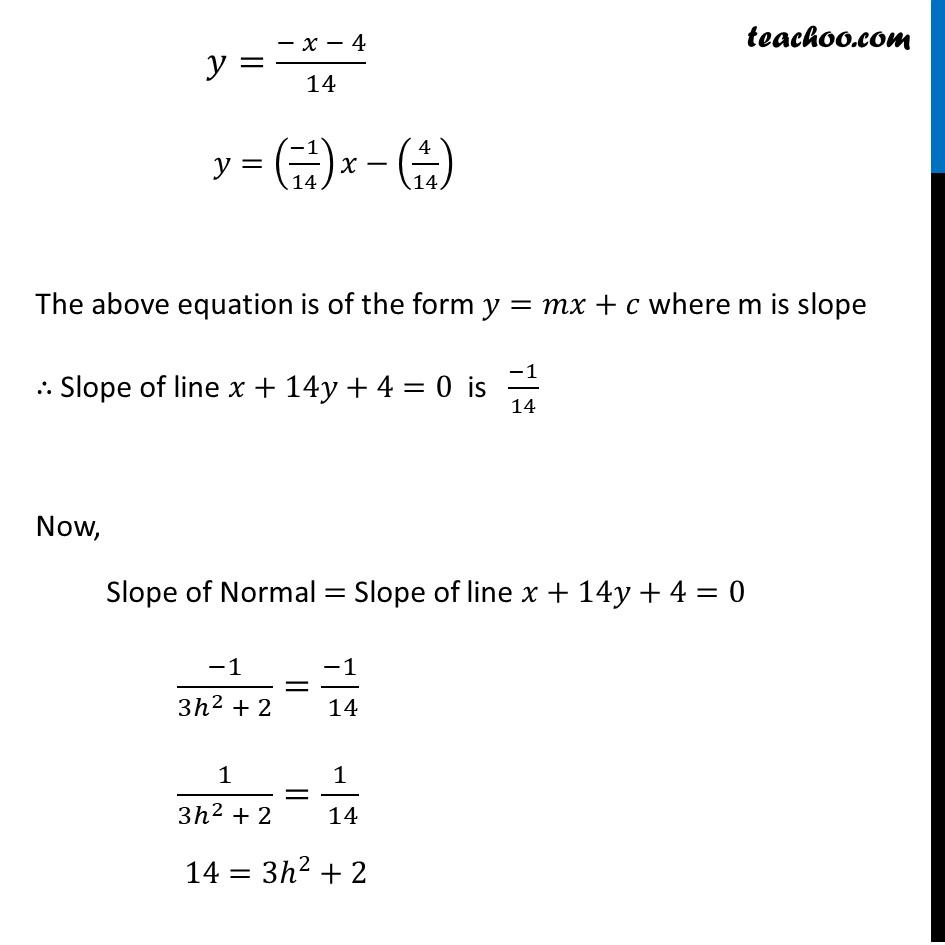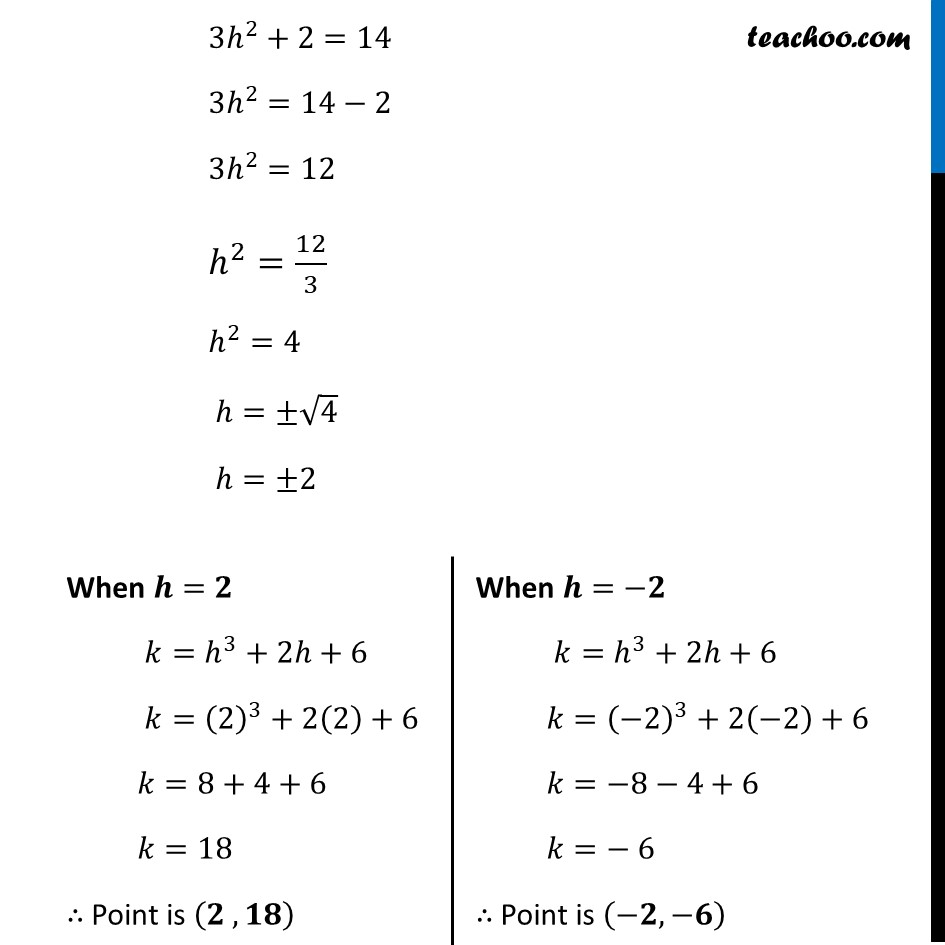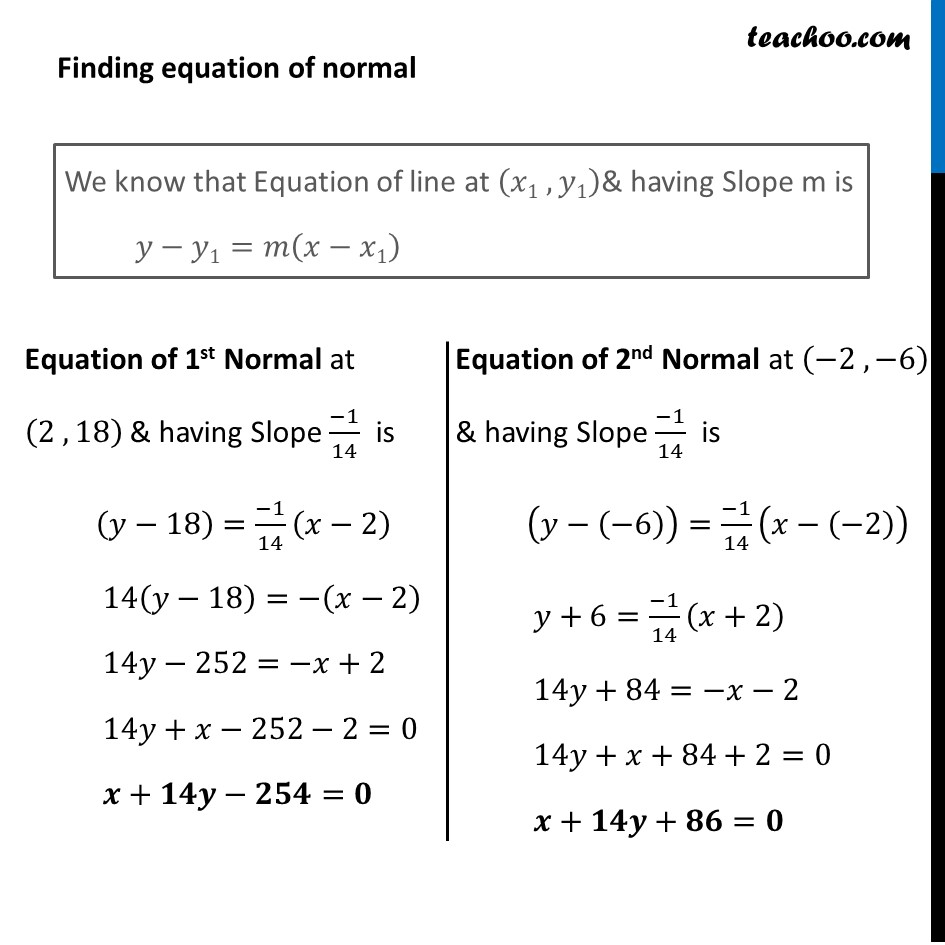Learn in your speed, with individual attention - Teachoo Maths 1-on-1 Class

### Transcript

Question 21 Find the equation of the normal to the curve 𝑦=𝑥^3+2𝑥+6 which are parallel to the line 𝑥+14𝑦+4=0.Let (ℎ , 𝑘) be the point on the Curve at which Normal is to be taken Given Curve is 𝑦=𝑥^3+2𝑥+6 Since point (ℎ , 𝑘) is on the Curve ∴ (ℎ , 𝑘) will satisfies the Equation of Curve Putting 𝑥=ℎ , 𝑦=𝑘 𝑘=ℎ^3+2ℎ+6 We know that Slope of a tangent to the Curve is 𝑑𝑦/𝑑𝑥 𝑦=𝑥^3+2𝑥+6 Differentiating w.r.t. 𝑥 𝑑𝑦/𝑑𝑥=3𝑥^2+2 Since tangent to be taken from (ℎ , 𝑘) Slope of tangent at (ℎ , 𝑘) is 〖𝑑𝑦/𝑑𝑥│〗_((ℎ, 𝑘) )=3ℎ^2+2 We know that Slope of tangent × Slope of Normal =−1 (3ℎ^2+2) × Slope of Normal =−1 Slope of Normal = (−1)/(3ℎ^2 + 2) Also, Given that Normal is parallel to the line 𝑥+14𝑦+4=0 If two lines are parallel then slopes are equal ⇒ Slopes of Normal = Slope of line 𝑥+14𝑦+4=0 Now, line is 𝑥+14𝑦+4=0 14𝑦=−𝑥−4 𝑦=(− 𝑥 − 4)/14 𝑦=((−1)/14)𝑥−(4/14) The above equation is of the form 𝑦=𝑚𝑥+𝑐 where m is slope ∴ Slope of line 𝑥+14𝑦+4=0 is (−1)/14 Now, Slope of Normal = Slope of line 𝑥+14𝑦+4=0 (−1)/(3ℎ^2 + 2)=(−1)/( 14) 1/(3ℎ^2 + 2)=1/( 14) 14=3ℎ^2+2 3ℎ^2+2=14 3ℎ^2=14−2 3ℎ^2=12 ℎ^2=12/3 ℎ^2=4 ℎ=±√4 ℎ=±2 When 𝒉=𝟐 𝑘=ℎ^3+2ℎ+6 𝑘=(2)^3+2(2)+6 𝑘=8+4+6 𝑘=18 ∴ Point is (𝟐 , 𝟏𝟖) When 𝒉=−𝟐 𝑘=ℎ^3+2ℎ+6 𝑘=(−2)^3+2(−2)+6 𝑘=−8−4+6 𝑘=− 6 ∴ Point is (−𝟐, −𝟔) Finding equation of normal We know that Equation of line at (𝑥1 , 𝑦1)& having Slope m is 𝑦−𝑦1=𝑚(𝑥−𝑥1) Equation of 1st Normal at (2 , 18) & having Slope (−1)/14 is (𝑦−18)=(−1)/14 (𝑥−2) 14(𝑦−18)=−(𝑥−2) 14𝑦−252=−𝑥+2 14𝑦+𝑥−252−2=0 𝒙+𝟏𝟒𝒚−𝟐𝟓𝟒=𝟎 Equation of 2nd Normal at (−2 , −6) & having Slope (−1)/14 is (𝑦−(−6))=(−1)/14 (𝑥−(−2)) 𝑦+6=(−1)/14 (𝑥+2) 14𝑦+84=−𝑥−2 14𝑦+𝑥+84+2=0 𝒙+𝟏𝟒𝒚+𝟖𝟔=𝟎